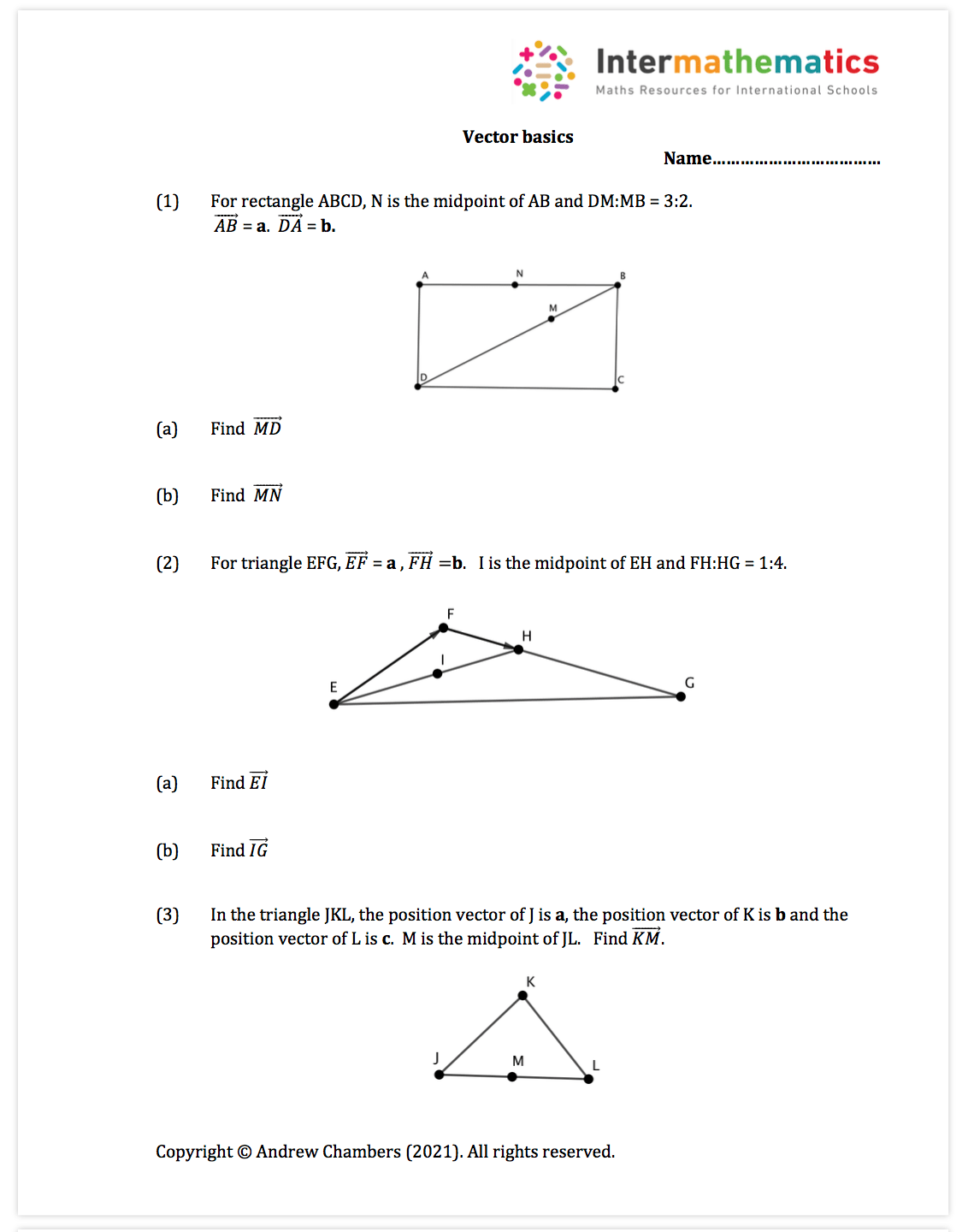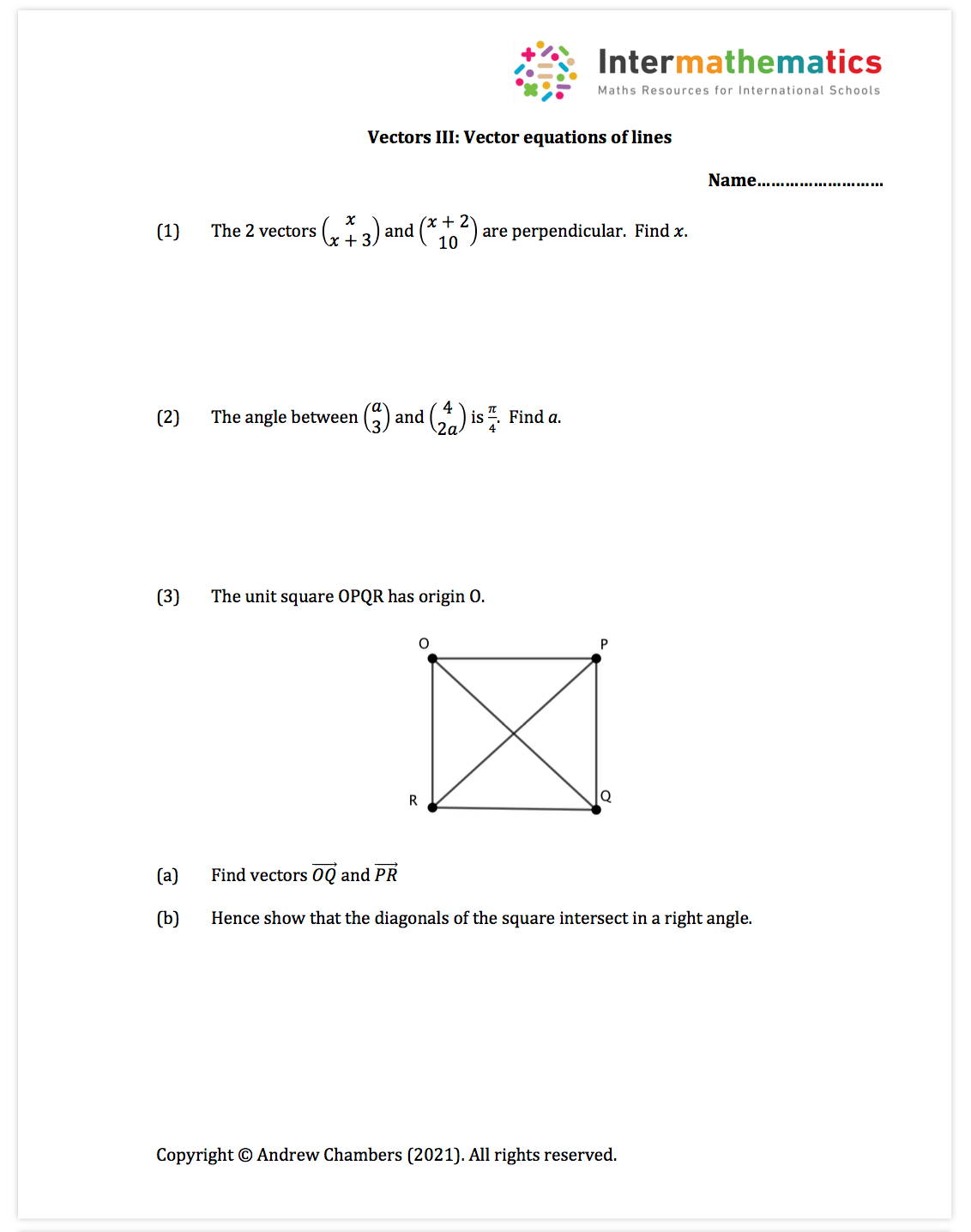# Vectors Free Content

Vectors Content: To support the teaching of vector geometry, vector equations of lines and planes, intersection of line, intersection of planes, angles between lines and lines and planes.

Worksheets in premium set: 9 worksheets (around 20 pdf pages of questions and around 35 pdf pages of fully typed solutions)

I have used Geogebra extensively in this unit to help visualize the problems and solutions.

Vectors worksheet example:Vectors worksheet example:Vectors worksheet example: